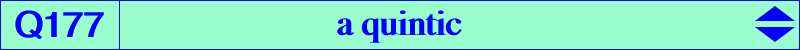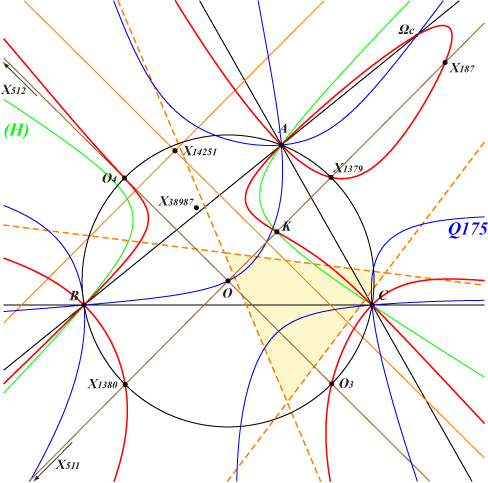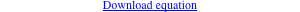too complicated to be written here. Click on the link to download a text file.X(6), X(187), X(511), X(512), X(1379), X(1380) infinite points of K024 Ωa, Ωb, Ωc : centers of the Apollonius circles O3, O4 : on the line {3,512} and on the circumcircle P1, P2 : on the line {3,512} and on the circum-hyperbola (H) with center X(38987), perspector X(3569) Z on K003, see Q175 further details belowQ177 is a quintic analogous to Q175. It has five real asymptotes : • two are the parallel and the perpendicular at X(14251) to the Brocard axis. • three are parallel to the asymptotes of the cubic K024 and to the sidelines of the CircumNormal triangle with vertices on (O) and K003. A, B, C are nodes on Q177 with nodal tangents parallel and perpendicular to the Brocard axis. The remaining points on the sidelines of ABC are Ωa, Ωb, Ωc on the Lemoine axis which also passes through X(187) and X(512) on Q177. Q177 meets the circumcircle (O) at X(1379), X(1380), O3, O4 which are obviously the vertices of a square. The points P1, P2 mentioned above are not always real and their midpoint (always real) is the barycentric quotient X(351) ÷ X(230), SEARCH = 9.60075856465753. The hyperbola (H) passes through {6, 232, 250, 262, 264, 325, 511, 523, 842, 1485, 2065, etc}.